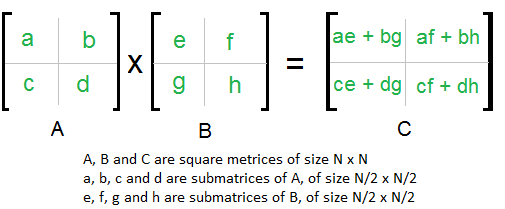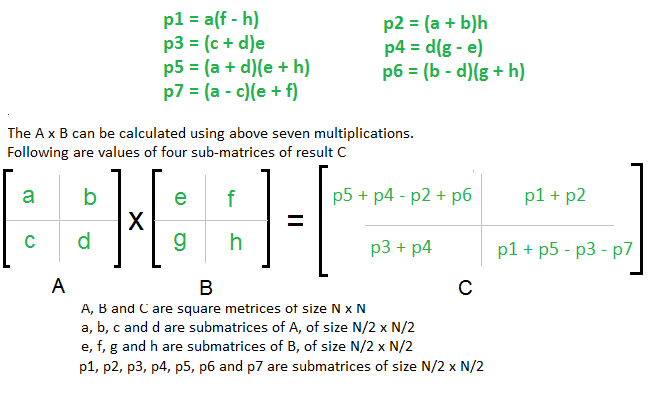# Divide and Conquer | Set 5 (Strassen’s Matrix Multiplication)

Given two square matrices A and B of size n x n each, find their multiplication matrix.

Naive Method
Following is a simple way to multiply two matrices.

 `void` `multiply(``int` `A[][N], ``int` `B[][N], ``int` `C[][N]) ` `{ ` `    ``for` `(``int` `i = 0; i < N; i++) ` `    ``{ ` `        ``for` `(``int` `j = 0; j < N; j++) ` `        ``{ ` `            ``C[i][j] = 0; ` `            ``for` `(``int` `k = 0; k < N; k++) ` `            ``{ ` `                ``C[i][j] += A[i][k]*B[k][j]; ` `            ``} ` `        ``} ` `    ``} ` `} `

Time Complexity of above method is O(N3).

Divide and Conquer
Following is simple Divide and Conquer method to multiply two square matrices.
1) Divide matrices A and B in 4 sub-matrices of size N/2 x N/2 as shown in the below diagram.
2) Calculate following values recursively. ae + bg, af + bh, ce + dg and cf + dh.In the above method, we do 8 multiplications for matrices of size N/2 x N/2 and 4 additions. Addition of two matrices takes O(N2) time. So the time complexity can be written as

```T(N) = 8T(N/2) + O(N2)

From Master's Theorem, time complexity of above method is O(N3)
which is unfortunately same as the above naive method.
```

Simple Divide and Conquer also leads to O(N3), can there be a better way?
In the above divide and conquer method, the main component for high time complexity is 8 recursive calls. The idea of Strassen’s method is to reduce the number of recursive calls to 7. Strassen’s method is similar to above simple divide and conquer method in the sense that this method also divide matrices to sub-matrices of size N/2 x N/2 as shown in the above diagram, but in Strassen’s method, the four sub-matrices of result are calculated using following formulae.Time Complexity of Strassen’s Method
Addition and Subtraction of two matrices takes O(N2) time. So time complexity can be written as

```T(N) = 7T(N/2) +  O(N2)

From Master's Theorem, time complexity of above method is
O(NLog7) which is approximately O(N2.8074)
```

Generally Strassen’s Method is not preferred for practical applications for following reasons.
1) The constants used in Strassen’s method are high and for a typical application Naive method works better.
2) For Sparse matrices, there are better methods especially designed for them.
3) The submatrices in recursion take extra space.
4) Because of the limited precision of computer arithmetic on noninteger values, larger errors accumulate in Strassen’s algorithm than in Naive Method (Source: CLRS Book)

Easy way to remember Strassen’s Matrix Equation